# Round

This chapter illustrates three functions to round numbers in Excel. ROUND, ROUNDUP and ROUNDDOWN.

Before your start: if you round a number, you lose precision. If you don't want this, show fewer decimal places without changing the number itself.

### Round

The ROUND function in Excel rounds a number to a specified number of digits. The ROUND function rounds up or down. 1, 2, 3 and 4 get rounded down. 5, 6, 7, 8 and 9 get rounded up.

1. For example, round a number to three decimal places.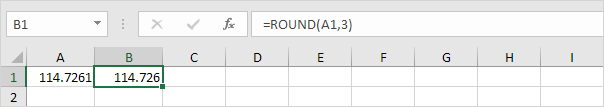Note: 114.7261, 114.7262, 114.7263 and 114.7264 get rounded down to 114.726 and 114.7265, 114.7266, 114.7267, 114.7268 and 114.7269 get rounded up to 114.727.

2. Round a number to two decimal places.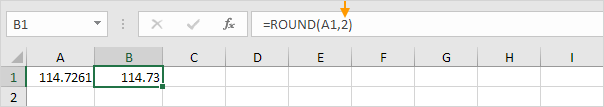3. Round a number to one decimal place.4. Round a number to the nearest integer.5. Round a number to the nearest 10.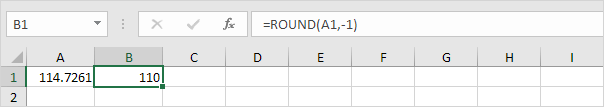6. Round a number to the nearest 100.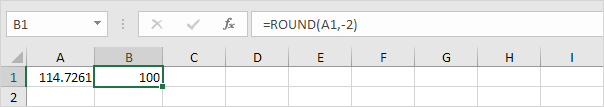7. Round a number to the nearest 1000.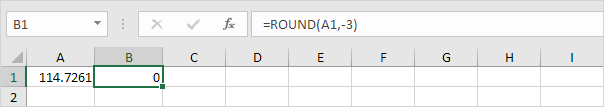8. Round a negative number to one decimal place.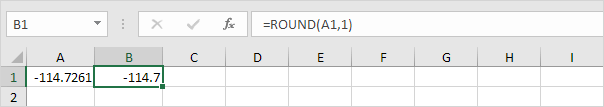9. Round a negative number to the nearest integer.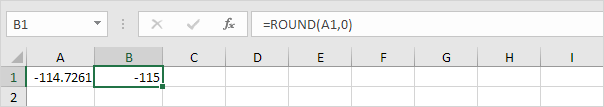### RoundUp

The ROUNDUP function in Excel always rounds a number up (away from zero). 1, 2, 3, 4, 5, 6, 7, 8 and 9 get rounded up.

1. For example, round a number up to three decimal places.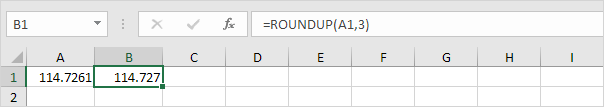Note: 114.7261, 114.7262, 114.7263, 114.7264, 114.7265, 114.7266, 114.7267, 114.7268 and 114.7269 get rounded up to 114.727.

2. Round a number up to two decimal places.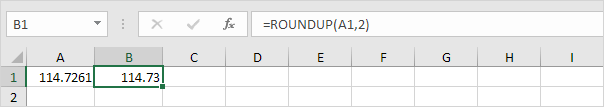3. Round a number up to one decimal place.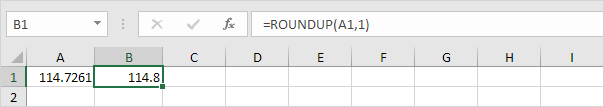4. Round a number up to the nearest integer.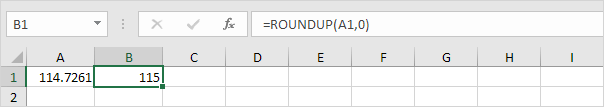5. Round a number up to the nearest 10.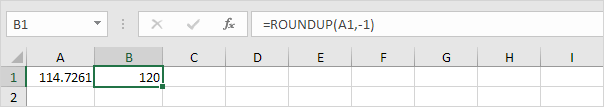6. Round a number up to the nearest 100.7. Round a number up to the nearest 1000.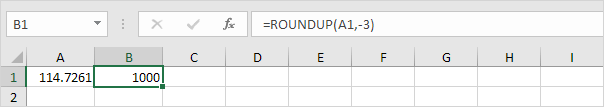8. Round a negative number up to one decimal place.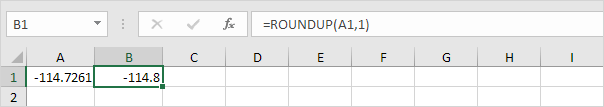Note: remember, the ROUNDUP function rounds a number up (away from zero).

9. Round a negative number up to the nearest integer.Note: again, the ROUNDUP function rounds a number up (away from zero).

### RoundDown

The ROUNDDOWN function in Excel always rounds a number down (toward zero). 1, 2, 3, 4, 5, 6, 7, 8 and 9 get rounded down.

1. For example, round a number down to three decimal places.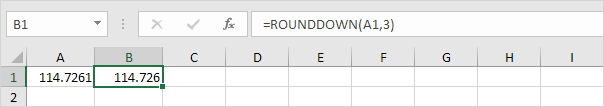Note: 114.7261, 114.7262, 114.7263, 114.7264, 114.7265, 114.7266, 114.7267, 114.7268 and 114.7269 get rounded down to 114.726.

2. Round a number down to two decimal places.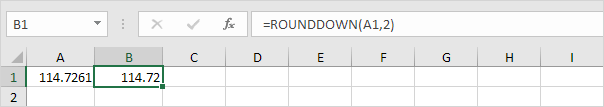3. Round a number down to one decimal place.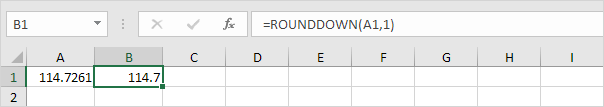4. Round a number down to the nearest integer.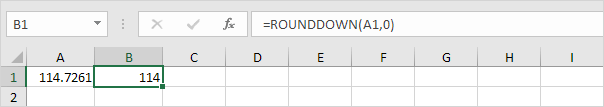5. Round a number down to the nearest 10.6. Round a number down to the nearest 100.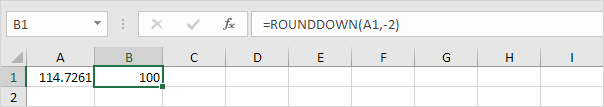7. Round a number down to the nearest 1000.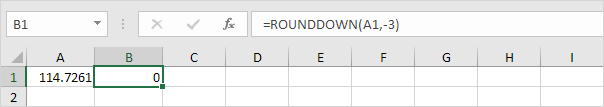8. Round a negative number down to one decimal place.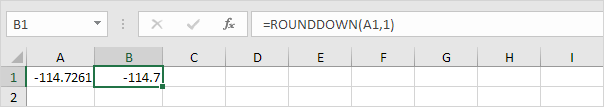Note: remember, the ROUNDDOWN function rounds a number down (toward zero).

9. Round a negative number down to the nearest integer.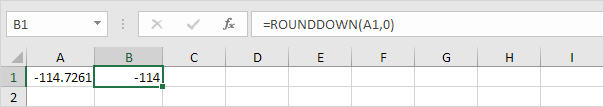Note: again, the ROUNDDOWN function rounds a number down (toward zero).

Go to Next Chapter: Formula Errors